Ready to present your report but at the last minute Excel formula giving wrong answer or not updating?

You are not alone!

In this article, we will cover 3 reasons why your formula may not be working properly and how to troubleshoot Excel formula:

Download this Excel workbook so you can practice along with us:

### 1. Calculation is configured to “Manual”

Suppose you have a worksheet calculating the total expenses and incomes.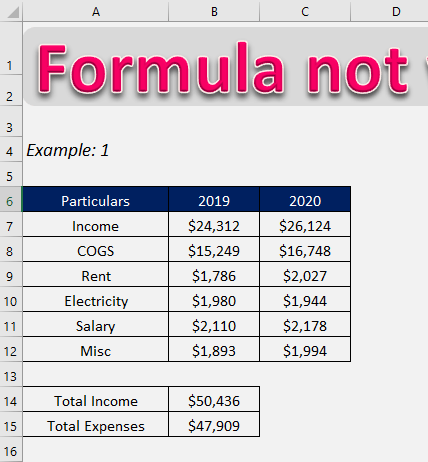Say, you update a value in the source data. i.e. electricity for the year 2020 changed from \$1944 to \$2210. You notice that the total expense contains old values, even though the source data for the formula-bearing cells have been changed.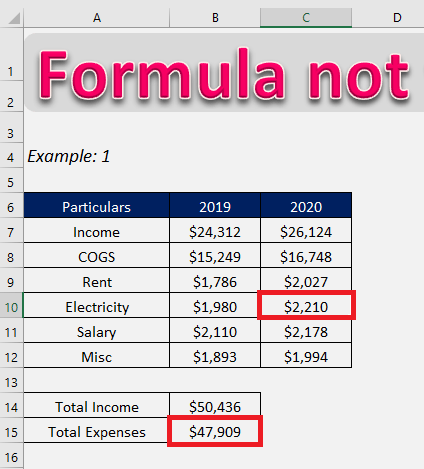This means that your formulas are not updating automatically as and when the source data is changing. It is a simple settings issue why Excel formula giving wrong answers and you can easily fix it

The reason that Excel is not updating your formulas is the calculation method of Excel has been changed from Automatic to Manual. There are two ways to correct this:

Method 1: Change Formula setting back to Automatic

STEP 1: Select the File tab.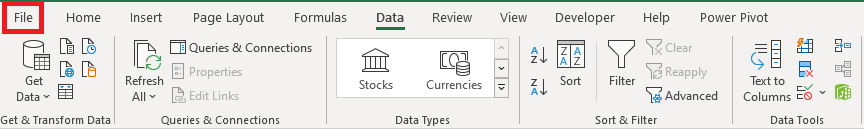STEP 2: Click Options from the menu on the left side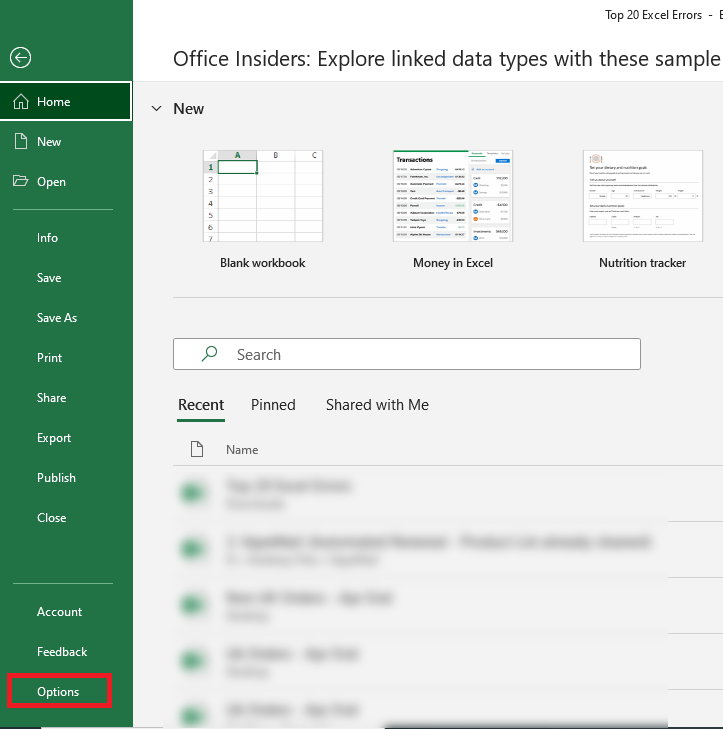STEP 3: In the dialog box, click on Formulas from the left panel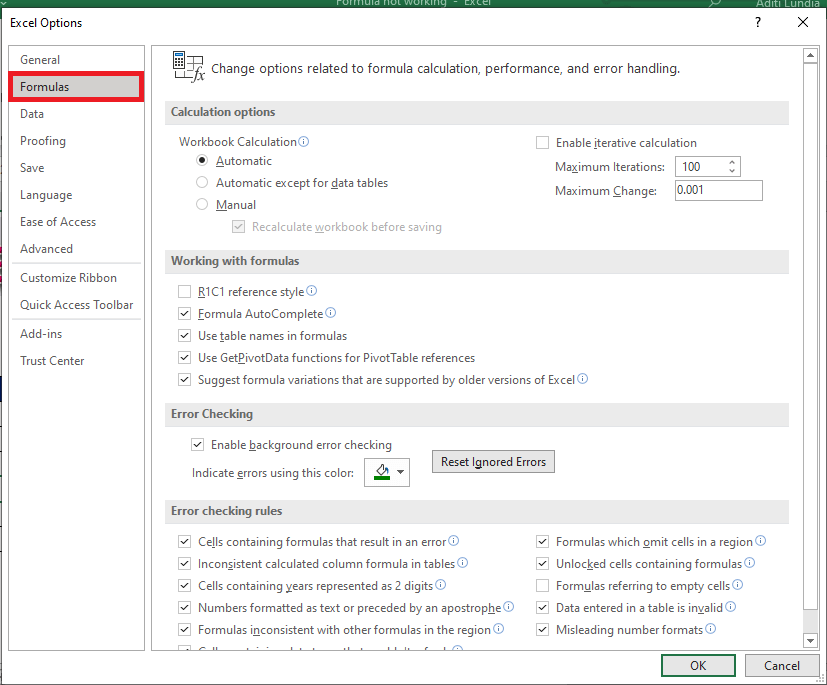STEP 4: Under the Calculation options section, select Automatic under Workbook Calculation.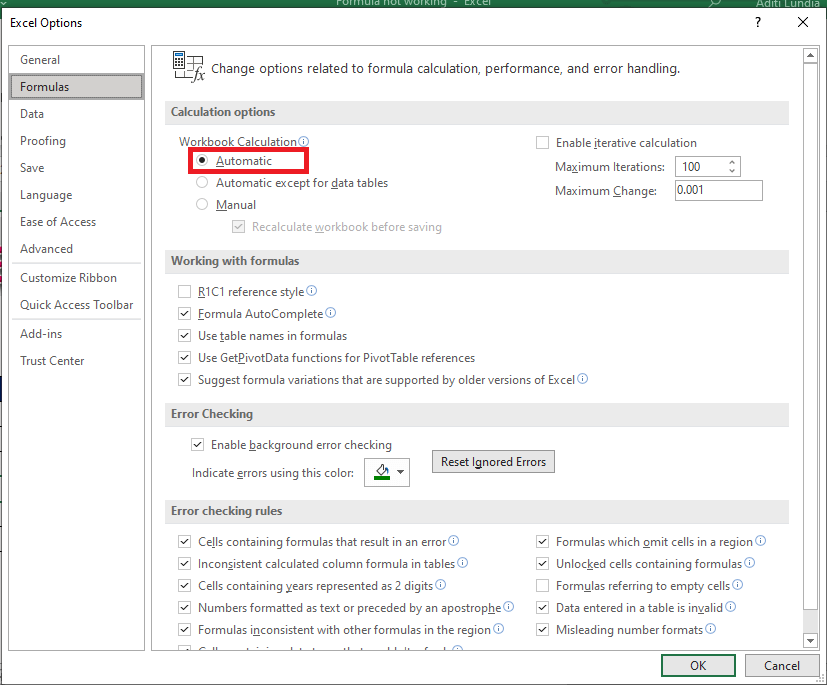Or, you could simply go to the Formulas tab > Calculation group, click the Calculation Options button, and select Automatic.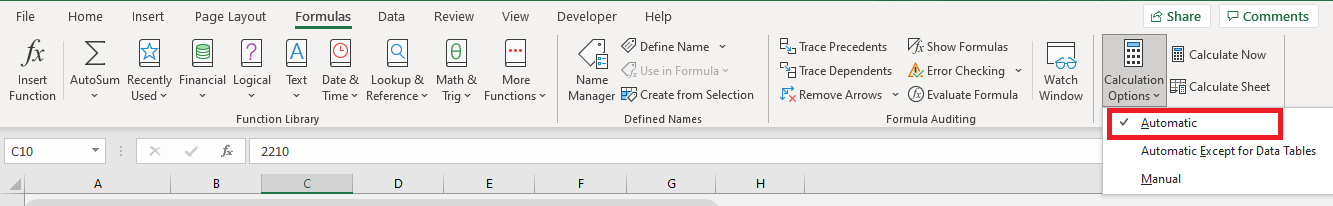After this, your formulas will be calculated as and when the dependant cell values are changed.

Method 2: Recalculate the Entire Sheet

You can force Excel to recalculate the entire sheet or workbook by the following steps. To recalculate the entire workbook:

Click the Calculate Now button on the Formulas tab > Calculation group.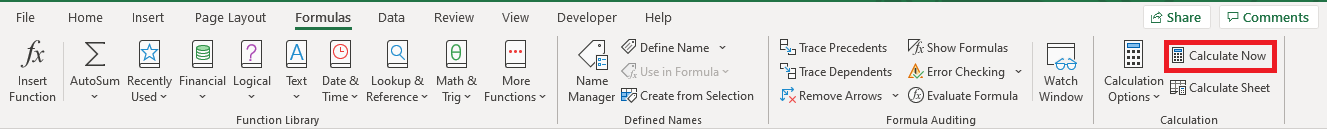You could simply press F9 to recalculate the entire worksheet and Shift + F9 to recalculate the active worksheet only.

This will force Excel to recalculate all the formulas in the active worksheet or the entire workbook, as the case may be.

### 2. Show Formula button is turned on

If your formula is displaying the function instead of the calculated result, it is because the Show Formula mode is turned on.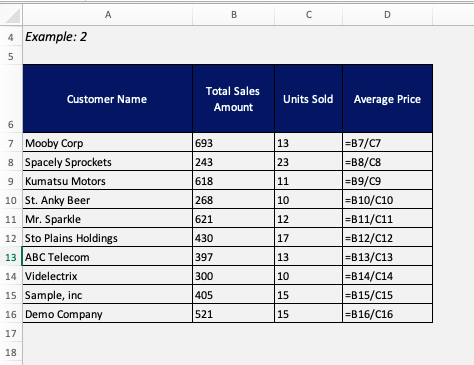To turn it off:

Go to Formula Tab > Under Formula Auditing group, Click Show Formulas.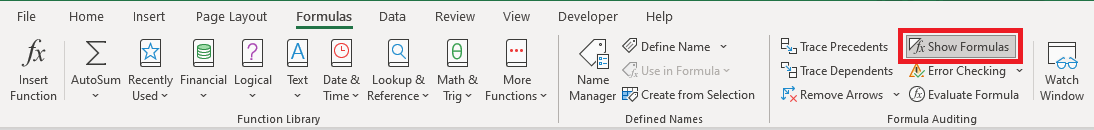Or, Press Ctrl + `

This will turn off the show formula mode and show the calculated result!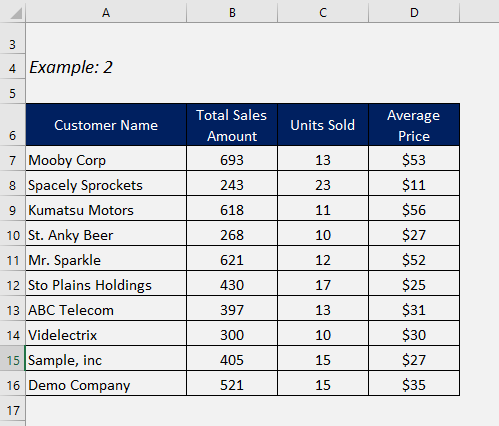### 3. Formula is stored as text

Even after turning show formula mode off, the cell is displaying function as shown in the image below: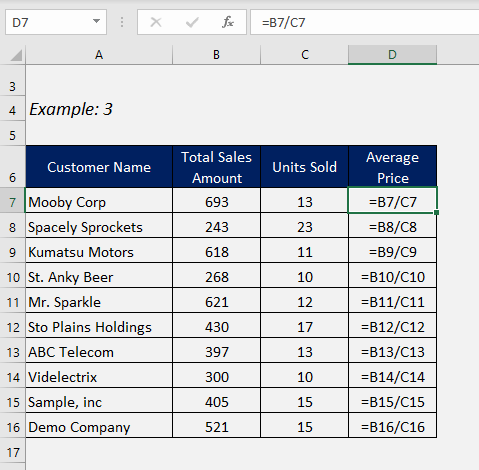This is because your formula is stored as text.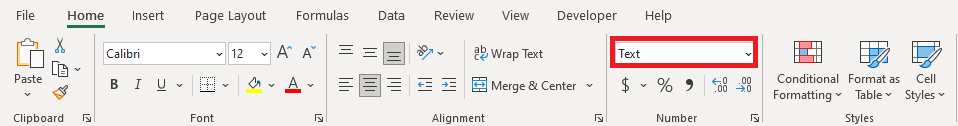To change it, go to Home tab> Select General from the dropdown.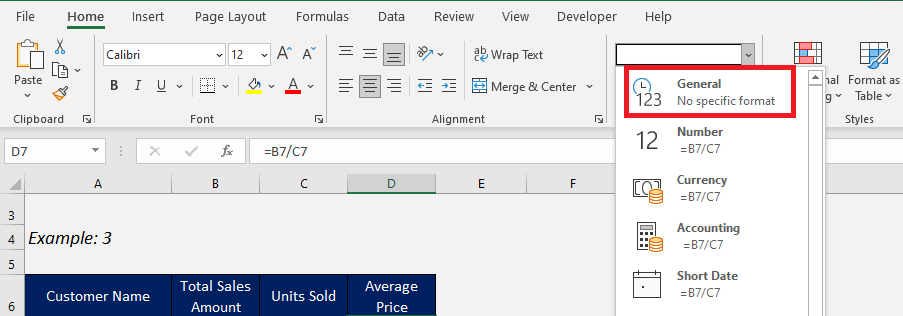Then, press F2 and then Enter to recalculate the value in the cell. Your calculated result will now be displayed!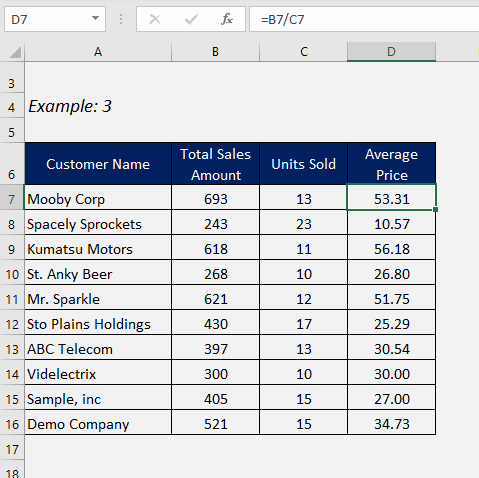### Conclusion

So now it must be clear that Excel was not automatically recalculating your formulas due to a settings issue and we hope that after watching this Excel troubleshoot formula tutorial, your problem has been solved and you can continue with smooth functioning on Excel!